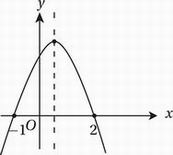# SAT Math Multiple Choice Question 682: Answer and Explanation

### Test Information

Question: 682

7. The value of y varies with x according to the equation y = a(x - 2)(x + 1), where a < 0. As the value of x increases from 0 to 5, which of the following best describes the behavior of y?

• A. It increases and then decreases.
• B. It decreases and then increases.
• C. It increases only.
• D. It decreases only.

Explanation:

A